# Arrays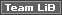We first encountered arrays in Chapter 4 where we looked at what an array is, and the difference between static and dynamic arrays. You will remember that an array is simply a sequential set of data elements, all with the same name and data type, referenced by an index. We declare an array by placing parentheses after the variable name . If we know how many elements we want the array to contain, we can specify this when we create the array, the number indicates the upper bound of the array:

` Dim intArray(2) As Integer     'Declares an array of 3 integers `

In the example above, the bounds ( To 2 ) indicate that the array will have three elements, intArray(0) , intArray(1) , and intArray(2) .

 Important Remember, in VBA all arrays are indexed from upwards by default. If you want to override this behavior and make the first element of your array have an index of 1 , you would put an Option Base 1 statement in the Declarations section of the module. Option Compare DatabaseOption ExplicitOption Base 1

We can declare what is known as a dynamic array by omitting the bounds. We should do this if we do not know how many elements the array will contain, or if the array will need to be resized later:

` Dim intArray() As Integer     'Declares a dynamic array of integers `

Before using a dynamic array, but after declaring it, we always need to tell VBA how many elements the array should contain by using a ReDim command:

` ReDim intArray(2)             'Resizes the array to hold 3 elements `

### Multi-dimensional Arrays

So far, all the arrays that we have been using have been one-dimensional. However, we might wish to store data that relates to a position on a grid, map, or mathematical settings like matrices. Arrays can have two, three or up to 60 dimensions and can store information in this way. For instance, we could store the SalesID and CompanyID of a number of sales in a 2 x 3 array like this:

SalesID

CompanyID

1

6

2

4

4

9

To declare a multi-dimensional array, like the one above, simply specify the bounds of each dimension separated by commas: note that we specify the columns and then the rows. For example, to specify an array of 2 ( columns ) x 3 (rows), for instance, 6 elements whose dimensions start at 1, use the following syntax:

` Dim intNum(1 To 2, 1 To 3) As Integer `

Alternatively, for an array of the same size , but whose dimensions start at 0, you could use:

` Dim intNum(1, 2) As Integer `

This would have the same effect as using this code:

` Dim intNum(0 To 1, 0 To 2) As Integer `

From another perspective, if we wanted to specify an array of 6 elements that had 3 columns and 2 rows (and not 2 columns and 3 rows as above), then we write the above styled declaration as:

` Dim intNum(0 To 2, 0 To 1) As Integer `

#### Dynamic Multi-dimensional Arrays

As with normal one-dimensional arrays, there is the option to make the arrays dynamic (that is, resizeable), according to our needs. To declare a dynamic, multi-dimensional array, we would use the following syntax:

` Dim intNum() As Integer Redim intNum(1 To 2, 1 To 3) `

Or, alternatively, for a dynamic array whose dimensions start at 0, we could use:

` Dim intNum() As Integer Redim intNum(1, 2) `

#### Referencing Elements in a Multi-dimensional Array

To reference elements in a multi-dimensional array, we simply specify the appropriate number of indexes to the array. The following code displays the results of raising the numbers 2 and 3 to the 3rd, 4th, and 5th power in turn . In other words, it displays the values of 2 3 , 2 4 , 2 5 , 3 3 , 3 4 , and 3 5 . We do this by using the exponent operator ( ^ ):

`   Sub MultiDimArray()     Dim i As Integer     Dim j As Integer     Dim intNum() As Integer                   'Create a dynamic array     ReDim intNum(2 To 3, 3 To 5)              'Resize the array     For i = 2 To 3                            'Populate the array     For j = 3 To 5     intNum(i, j) = i ^ j     Next j     Next i     For i = 2 To 3                            'Print the contents...     For j = 3 To 5                          '...of the array     Debug.Print i & "^" & j & "=" & intNum(i, j)     Next j     Next i     End Sub   `

This procedure produces the following results: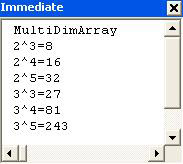As you can see, the procedure has two parts : the calculation and then printing the results. Each part has two loops , one nested inside the other. The inside loop is executed three times ( For j = 3 to 5 ) for each value of i in the outer loop, which is executed twice ( For i = 2 to 3 ).

The number of elements in a multi-dimensional array (that is, the number of separate values that it can hold) is calculated by multiplying together the number of elements in each dimension of the array. For example, the array in the procedure above would be able to hold 2 x 3 = 6 values.

Similarly, the following declaration:

` Dim intNum() As Integer ReDim intNum(9, 19, 29) `

would produce an array of 10 x 20 x 30 = 6000 elements ( assuming there is no Option Base 1 statement in the module).

### Memory Considerations

We mentioned in Chapter 3 that it's important to select the right data type for your variables . This helps to avoid errors, but it's also important because the different data types take up different amounts of memory. For example, a long integer takes up more memory than an integer.

Arrays require twenty bytes of memory, plus four bytes for each array dimension, plus the number of bytes occupied by the data itself. The memory occupied by the data can be calculated by multiplying the number of data elements by the size of each element.

Therefore, to calculate the memory that the integer array intNum(9, 19, 29) would take up, we multiply the number of elements in the array by the size of each of the elements:

` 10 x 20 x 30 = 6,000 elements 6000 x 2 bytes for an integer = 12,000 bytes `

Then add the overhead, which is always equal to 20 bytes + 4 bytes per dimension:

` 20 bytes + (3 x 4 bytes) = 32 bytes `

This gives a total of 12,032 bytes.

If we compare this to the amount of memory that the array would have taken up if it had been declared as a Variant , you'll see just why it is important to choose your data type carefully .

` Dim varName As Variant ReDim varName(9, 19, 29) `

Variant type variables containing strings require (22 + the string length) bytes of memory per element. So, the memory requirements would have been:

` 10 x 20 x 30 = 6,000 elements 6000 x 22 bytes (minimum) for a Variant = 132,000 bytes `

` 20 bytes + (3 x 4 bytes) = 32 bytes `

This gives a total of at least 132,032 bytes - around 128K.

It is clear that the more dimensions you have in your array, the number of elements in each dimension, and the larger the data type, the easier it is to consume vast amounts of memory. Computer memory is a precious resource and like money you never seem to have enough of it, hence choose the data type of the right size depending on the application at hand.

In theory, the maximum number of dimensions that you can declare in an array is 60, as we noted earlier. In practice, though, you will probably find it very hard to keep track of what is happening in arrays of more than three, or perhaps four, dimensions.

#### Erasing Arrays

When an array's lifetime expires , the memory that the array variable was taking up is automatically reclaimed by VBA. So, if you declare an array at the procedure level, then when the array is destroyed at the end of the procedure, VBA reclaims any memory that it was taking up.

However, you might want to explicitly free up the memory that an array was taking up without actually destroying the variable itself. For example, you might be using a module-level array variable (for instance, a variable declared in the Declaration section of a standard code module). Because standard code modules are always loaded into memory in Access, the array variable will only be destroyed when Access is closed down.

If, in the meantime, you want to "empty" the array and free up the memory that its contents were taking up, then you can use the Erase statement. If you use the Erase statement on a dynamic array, that's just what happens - we free up memory:

` Erase intNum       'Empties contents of intNum array and reclaims its memory `

However, this only works with dynamic arrays. Using the Erase statement on a static array will reinitialize the array, but will not reclaim the memory that it takes up. So, if you only need to use an array for part of the time - especially if it has a long lifetime - you should consider declaring it as a dynamic array.

When we say that an array is reinitialized, we mean that its elements are restored to their initial values. For numeric variables, the initial value is 0, for strings the initial value is an empty string ("") and for variants the initial value is the special Empty value.

As we can see, the Erase statement has different effects on different types of arrays, and it even has different effects on different types of static arrays as the following table illustrates:

Array Type

Using Erase on static array elements

Static numeric

Each element is set to zero

Static string (variable length)

Each element is set to zero length (that is "") string

Static string (fixed length)

Each element is set to zero

Static variant

Each element is set to Empty

Boolean

Each element is set to False

UDT ( User Defined Types)

Each element is set to its default

Objects

Each element is set to Nothing

### Parameter Arrays

VBA also allows you to pass parameter arrays to functions and sub procedures. A parameter array, as its name suggests, is an array of parameters: it is an Optional array of Variant type elements. In other words, a parameter array allows you to pass a variable number of arguments to a procedure. It can be useful if, at design-time, you don't know how many arguments you will want to pass to a procedure. Have a look at the following code:

`   Function Avge(ParamArray aValues() As Variant) As Double     Dim varValue As Variant     Dim dblTotal As Double     For Each varValue In aValues     dblTotal = dblTotal + varValue     Next     Avge = dblTotal / (UBound(aValues) + 1)     End Function   `

This function returns the average value of a series of numbers. If you add this procedure to a code module and use the Immediate window to determine the average of a series of numbers, you should see something like this when you hit the Enter key: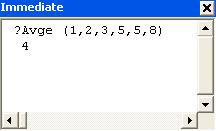In the above example, aValues() is a parameter array. To declare an argument as a parameter array, you just prefix it with the keyword ParamArray . There are a few important things to remember when declaring parameter arrays:

• A ParamArray argument may only appear as the last argument passed to a Sub or Function.

• ParamArray arguments are of type Variant.

• ParamArray arguments may not be passed as Optional , ByVal , or ByRef (as we said before a ParamArray is itself an Optional Variant Array).

• A ParamArray may not appear with an Optional argument in the same list of arguments; however, it may be preceded, in an argument list, by other arguments that are passed ByRef and ByVal .

In the Avge function, we loop through each of the elements in the aValues() array and add it to a running total, dblTotal .

` For Each varValue In aValues   dblTotal = dblTotal + varValue Next `

We then divide the total by the number of elements in the array.

` Avge = dblTotal / (UBound(aValues) + 1) `

We've used the UBound() function which, if you remember from Chapter 4, returns the value of the highest index in the array. Note that, here, we calculate the number of elements as UBound(aValues) + 1 . This is because parameter arrays always start at element 0 - even if you have specified Option Base 1 in the Declarations section of the module containing the procedure.

 Important That last sentence is important - if it didn't sink in just now, read it again. This is guaranteed to catch you out one day!

### The Array Function

If we have a series of values that we want to insert into an array, we can do so with the Array function. Look at the following subprocedure:

`   Sub MonthNames()     Dim varMonth As Variant     varMonth = Array("Jan", "Feb", "Mar", "Apr")     Debug.Print varMonth(1)     Debug.Print varMonth(2)     End Sub   `

If you were to execute this procedure, the values Feb and Mar would be displayed in the Immediate window.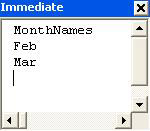The Array function accepts a comma-delimited list of values which it then returns as a one-dimensional array. Two things in particular are worth remembering when you use the Array function. Firstly - and somewhat counter-intuitively given the way parameter arrays work - the index of the first element in the returned variant array is determined by the current Option Base statement if there is one. In the example above, the code module had no Option Base statement, so the index of the first element of the array was . Hence, varMonth(1) contains Feb , the second element of the array. The second thing to remember is that the array returned by the Array function must be stored in a variable of type Variant .

Although conceptually different, in practice there is no difference between an array of Variant variables and a Variant variable containing an array.

### The GetRows() Method

Another way to use a Variant array is to use the GetRows() method of the Recordset object (refer to Chapter 6 if you want to freshen up on recordsets). This is used to copy a number of rows from a Recordset object into an array. The technique is very useful in a multi-user environment because it allows the Recordset object to be closed, minimizing potential locking conflicts, but still giving you access to the values in the records. In addition, it can be faster to perform repeated operations on the values stored in the array than continually re-reading the records from the Recordset object. That is because the array does not have the overhead of the sophisticated cursor functionality which Access provides via Recordset objects. Note, however, that because you will be working with a copy of the records, rather than the records themselves , any changes made to the values in the array will not be reflected in the recordset from which you copied them. So in practical terms, using this methodology is appropriate for tasks that are read-only, for example, analyzing last month's sales.

We'll now demonstrate with an example where we'll create an array which takes data from the first two rows of the Sales table.

### Try It Out-The GetRows() Method

1. Create a new code module in IceCream.mdb and call it Chapter 11 Code . Then type in the following code:

`   Sub TestGetRows()     Dim varValues As Variant     Dim recSales As Recordset     Dim intRowCount As Integer     Dim intFieldCount As Integer     Dim i As Integer     Dim j As Integer     Set recSales = CurrentDb().OpenRecordset("tblSales")     varValues = recSales.GetRows(2)     recSales.Close     intFieldCount = UBound(varValues, 1)     intRowCount = UBound(varValues, 2)     For j = 0 To intRowCount     For i = 0 To intFieldCount     Debug.Print "Row " & j & ", Field " & i & ": ";     Debug.Print varValues(i, j)     Next i     Next j     End Sub   `
2. Open the Immediate window and run TestGetRows . When you hit Enter , you should get a list of the contents of each field in each of the first two rows of the table tblSales .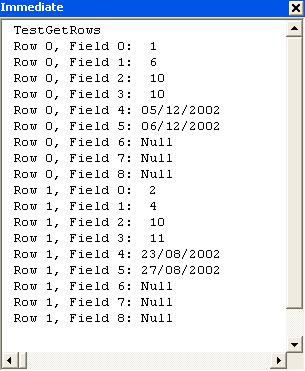How It Works

The GetRows() method takes as an argument the number of rows that we want to copy into the array:

` varValues = recSales.GetRows(2) `

Here, we copy the first two rows. The rows that are copied are relative to the current record. As the recordset has just been opened, the current record is the first row in the recordset, so the first two rows will be copied. If the number of rows requested is greater than the number of rows between the current record and the last record in the recordset, GetRows() will return all the available rows.

After the GetRows() method has been applied, the current row will be the one after those that have been copied. This is useful because it allows us to copy one block of records (say the first 50) into our array and process them. Then, when we have finished with them, we can read the next block.

Note that - as with the Array function - the rows are copied into a Variant variable, rather than into an array declared with the usual syntax.

` Dim varValues As Variant . . . varValues = recSales.GetRows(2) `

The array created by the GetRows() method is always two-dimensional. The first element corresponds to the field index, the second to the row index. To inspect the index of the last field returned, inspect the value of the highest index in the first dimension of the array:

` intFieldCount = UBound(varValues, 1) `

When using UBound() with a multi-dimensional array, you should specify which dimension you want to find the highest index. Specify it as a number following the array name.

To find the index of the last row returned, inspect the value of the highest index in the second dimension of the array returned:

` intRowCount = UBound(varValues, 2) `

Note that the array returned by GetRows() is zero-based . This means that the number of fields is intFieldCount+1 and the number of rows is intRowCount+1 .

Once we have determined the number of elements in each dimension of the array, we loop through each dimension, printing the results.

` For j = 0 To intRowCount   For i = 0 To intFieldCount     Debug.Print "Row " & j & ", Field " & i & ": ";     Debug.Print varValues(i, j)   Next i Next j `

Using a semicolon as a separator in the Immediate window causes the two expressions to be printed next to each other without a carriage return. So placing a semicolon at the end of the first Debug.Print line means that there will be no carriage return before the next line is printed. In other words, in the Immediate window, the output of the two code lines will be printed together on one line.

You can also use a comma to separate expressions in the Immediate window. This causes the two expressions to be printed next to each other, but separated by a tab (which sometimes makes the results easier to read).

### Detecting Arrays

We have just seen two different uses of Variant variables to hold arrays. Of course, one of the problems with using Variant variables to hold arrays is that it isn't obvious whether the variable contains an array or just contains a single value. For example, in the TestGetRows procedure above, the variable varValues only contained an array after the GetRows() method was used.

There are actually three different ways you can determine whether a variable contains a single value or an array. These involve using one of the following functions:

• IsArray()

• VarType()

• TypeName()

#### The IsArray() Function

This function returns True if the variable passed to it is an array, and False if it is not. Have a look at the following procedure:

`   Sub ArrayTest()     Dim intNum1 As Integer     Dim intNum(1 To 10) As Integer     Debug.Print "intnum1: " & IsArray(intNum1)     Debug.Print "intnum: " & IsArray(intNum)     End Sub   `

You can run this procedure by typing the above code into a module and then either hitting F5, or typing ArrayTest in the Immediate window and hitting Enter . Either way, you should see the words False and True appear in the Immediate window: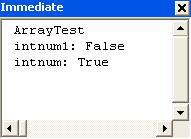This is because IsArray(intNum1) is False and IsArray(intNum) is True . In other words, intNum1 is not an array, whereas intNum is.

#### The VarType() Function

Another method for determining whether or not a variable is an array is to use the VarType() function. We looked at this in Chapter 3 (See Try It Out - Examining a Variant ) when we used it to determine the type of value being held within a variable of type Variant . In fact, we can also use this function to determine whether the variable holds an array. Have a look at this procedure:

`   Sub ArrayTest2()     Dim intNum1 As Integer     Dim intNum(1 To 10) As Integer     Debug.Print "intnum1: " & VarType(intNum1)     Debug.Print "intnum: " & VarType(intNum)     End Sub   `

If you run ArrayTest2 by typing the above code into a code module and hitting F5 , you should see the values 2 and 8194 in the Immediate window: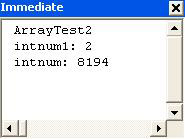Now, you are probably asking yourself "What on earth does 8194 mean?" Well, if you cast your mind back to our discussion of the VarType() function in Chapter 3: Sub VariantExample() in Try It Out - Examining a Varian t , you will remember that the subroutine prints out a number, via Debug.Print , indicating the type of data that the variable contains. The table below shows the values for each data type as well as the intrinsic constant that represents those numbers. For example, 2 or vbInteger indicates that the underlying data type of the variable is an integer.

Constant

Value

Variable type

vbEmpty

Empty ( uninitialized )

vbNull

1

Null

vbInteger

2

Integer

vbLong

3

Long Integer

vbSingle

4

Single

vbDouble

5

Double

vbCurrency

6

Currency

vbDate

7

Date

vbString

8

String

vbObject

9

Object

vbError

10

Error value

vbBoolean

11

Boolean

vbVariant

12

Variant

vbDataObject

13

Data access object

vbDecimal

14

Decimal value

vbByte

17

Byte

vbUserDefinedType

36

UDT (User Defined Type)

vbArray

8192

Array

As you can see from the table, arrays are denoted by the value 8192 ( vbArray ). In fact, vbArray is never returned on its own. If the variable passed to VarType() is an array, then the number that the VarType() function returns is a combination of vbArray and the underlying data type. So, in our example, both variables are of integer type ( vbInteger , or 2 ), and the second one is an array ( vbArray , or 8192 ) of integers, giving a total of vbArray + vbInteger = 8194 .

Because the number 8192 can be represented by the intrinsic constant vbArray , we can modify the procedure to make the results a little more readable:

`   Sub ArrayTest3()     Dim intNum1 As Integer     Dim intNum(1 To 10) As Integer     Debug.Print "Array: " & (VarType(intNum1) > vbArray),     Debug.Print "Type: " & (VarType(intNum1) And Not vbArray)     Debug.Print "Array: " & (VarType(intNum) > vbArray),     Debug.Print "Type: " & (VarType(intNum) And Not vbArray)     End Sub   `

If you run this procedure, you should get the results shown below: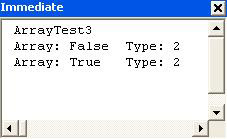The code looks a little more complex, but isn't that hard to follow. The first thing to remember is that putting parentheses around an expression in VBA forces the expression to be evaluated first. So the parentheses around (VarType(intNum) > vbArray) force that expression to be evaluated first. The result of this expression is True because VarType(intNum) (which equals 8194) is indeed greater than vbArray (8192).

The next step is to determine the data type of each variable. We do that with this expression:

` (VarType(intNum) And Not vbArray) `

The parentheses again cause the expression to be evaluated. But what exactly is being evaluated? To understand the logical expression And Not we need to start thinking in binary again. If you remember, in Chapter 3 we looked at how an integer variable could be used as a set of flags. Well, that's how the vbArray constant is used.

To see how this works, let's look at what VarType(intNum ) and vbArray look like in binary. We know from the previous procedure, ArrayTest2() , that the value of VarType(intNum ) is 8194 and that vbArray is 8192. In binary those are 10000000000010 and 10000000000000 respectively.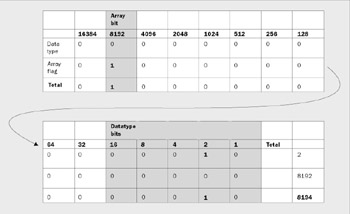The rightmost five binary digits are used to indicate the data type of the variable. The binary digit 2nd from the left on the top set, whose value is 8192, is used to flag whether the variable is an array or not. If it is an array, this flag is set to 1, increasing the value of the VarType by 8192.

Don't worry about the other 10 bits. They are reserved for use in future versions of VBA and don't hold any meaningful information for us.

What we want to do is to determine the value of the digits without the influence of the 14th digit, that is, the vbArray digit. To do this we use the logical operator And against Not vbArray . Not vbArray is the reverse of vbArray . In other words, the 0s become 1s and the 1s become 0s. The result of an And operation is that bit flags in the result are set to 1 only if the bit was 1 in both the numbers being compared. So using an And with Not vbArray has the result of leaving 15 of the bits in the result the same as they were in the original number, while ensuring that the 14th bit is set to 0.

As you can see, the result of this is 2, which is what we were expecting, indicating that the underlying data type is an integer.

#### The TypeName() Function

The TypeName() function does much the same as the VarType() function, except that it returns its result in plainer terms.

For example, the following procedure:

`   Sub ArrayTest4()     Dim intNum1 As Integer     Dim intNum(1 To 10) As Integer     Debug.Print "intnum1: " & TypeName(intNum1)     Debug.Print "intnum: " & TypeName(intNum)     End Sub   `

will give these results: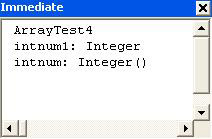As you can see, the return value of the TypeName() function is a lot easier to understand. It returns the type of the variable in plainer terms, Integer , and adds a pair of empty parentheses if the variable is an array, Integer() . However, if you need to detect programmatically whether a variable contains an array, you will find it easier to use either the IsArray() function or the VarType() function (which has the advantage over IsArray() in that it also returns the underlying data type.)

This concludes our look at arrays. Hopefully, splitting the discussion over two separate chapters hasn't confused you. This was necessary as we needed to discuss the fundamentals of what static and dynamic arrays were early in the book before we could move on to more unusual topics such as multi-dimensional arrays and using the GetRows() method to create an array. The next topic is unrelated to arrays, but is a continuation of another concept learned earlier in the book: passing arguments.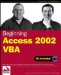Beginning Access 2002 VBA (Programmer to Programmer)
ISBN: 0764544020
EAN: 2147483647
Year: 2003
Pages: 256